## 几何变换的矩阵表示

### 二维仿射变换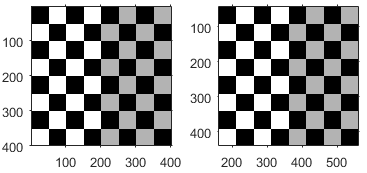$\left[\begin{array}{ccc}1& 0& {t}_{x}\\ 0& 1& {t}_{y}\\ 0& 0& 1\end{array}\right]$

tx 指定沿 x 轴的位移

ty 指定沿 y 轴的位移。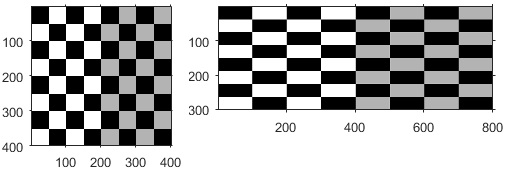$\left[\begin{array}{ccc}{s}_{x}& 0& 0\\ 0& {s}_{y}& 0\\ 0& 0& 1\end{array}\right]$

sx 指定沿 x 轴的缩放因子

sy 指定沿 y 轴的缩放因子。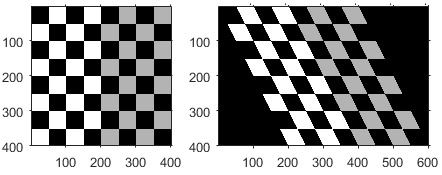$\left[\begin{array}{ccc}1& s{h}_{x}& 0\\ s{h}_{y}& 1& 0\\ 0& 0& 1\end{array}\right]$

shx 指定沿 x 轴的剪切因子。

shy 指定沿 y 轴的剪切因子。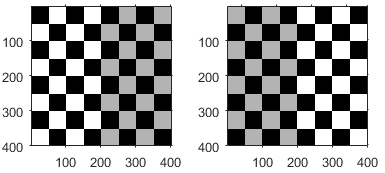$\left[\begin{array}{ccc}\text{cosd(2}\phi \text{)}& \text{sind(2}\phi \text{)}& 0\\ \text{sind(2}\phi \text{)}& -\text{cosd(2}\phi \text{)}& 0\\ 0& 0& 1\end{array}\right]$

φ 指定翻转轴的角度，以度为单位。

`[1 0 0; 0 -1 0; 0 0 1]`.

`[-1 0 0; 0 1 0; 0 0 1]`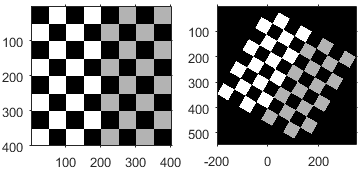$\left[\begin{array}{ccc}\mathrm{cosd}\left(\text{θ}\right)& -\mathrm{sind}\left(\text{θ}\right)& 0\\ \mathrm{sind}\left(\text{θ}\right)& \mathrm{cosd}\left(\text{θ}\right)& 0\\ 0& 0& 1\end{array}\right]$

θ 指定围绕原点的旋转角度（以度为单位）。

### 二维投影变换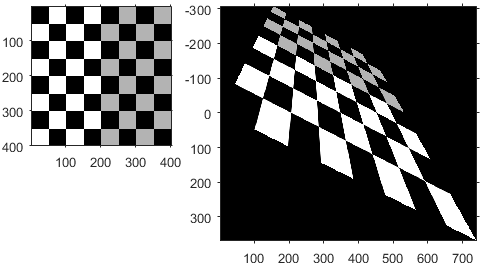`$\left[\begin{array}{ccc}1& 0& 0\\ 0& 1& 0\\ E& F& 1\end{array}\right]$`

E 和 F 对消失点有影响。

### 三维仿射变换

`$\left[\begin{array}{cccc}1& 0& 0& {t}_{x}\\ 0& 1& 0& {t}_{y}\\ 0& 0& 1& {t}_{z}\\ 0& 0& 0& 1\end{array}\right]$`

`$\left[\begin{array}{cccc}{s}_{x}& 0& 0& 0\\ 0& {s}_{y}& 0& 0\\ 0& 0& {s}_{z}& 0\\ 0& 0& 0& 1\end{array}\right]$`

`$\left[\begin{array}{cccc}1& 0& 0& 0\\ s{h}_{xy}& 1& 0& 0\\ s{h}_{xz}& 0& 1& 0\\ 0& 0& 0& 1\end{array}\right]$`

`$\begin{array}{l}x\text{'}=x\\ y\text{'}=y+s{h}_{xy}x\\ z\text{'}=z+s{h}_{xz}x\end{array}$`

`$\left[\begin{array}{cccc}1& s{h}_{yx}& 0& 0\\ 0& 1& 0& 0\\ 0& s{h}_{yz}& 1& 0\\ 0& 0& 0& 1\end{array}\right]$`

`$\begin{array}{l}x\text{'}=x+s{h}_{yx}y\\ y\text{'}=y\\ z\text{'}=z+s{h}_{yz}y\end{array}$`

`$\left[\begin{array}{cccc}1& 0& s{h}_{zx}& 0\\ 0& 1& s{h}_{zy}& 0\\ 0& 0& 1& 0\\ 0& 0& 0& 1\end{array}\right]$`

`$\begin{array}{l}x\text{'}=x+s{h}_{zx}z\\ y\text{'}=y+s{h}_{zy}z\\ z\text{'}=z\end{array}$`

`$\left[\begin{array}{cccc}-1& 0& 0& 0\\ 0& 1& 0& 0\\ 0& 0& 1& 0\\ 0& 0& 0& 1\end{array}\right]$`

`$\left[\begin{array}{cccc}1& 0& 0& 0\\ 0& -1& 0& 0\\ 0& 0& 1& 0\\ 0& 0& 0& 1\end{array}\right]$`

`$\left[\begin{array}{cccc}1& 0& 0& 0\\ 0& 1& 0& 0\\ 0& 0& -1& 0\\ 0& 0& 0& 1\end{array}\right]$`

`$\left[\begin{array}{cccc}1& 0& 0& 0\\ 0& \text{cosd}\left({\theta }_{x}\right)& -\text{sind}\left({\theta }_{x}\right)& 0\\ 0& \text{sind}\left({\theta }_{x}\right)& \text{cosd}\left({\theta }_{x}\right)& 0\\ 0& 0& 0& 1\end{array}\right]$`

`$\left[\begin{array}{cccc}\text{cosd}\left({\theta }_{y}\right)& 0& \text{sind}\left({\theta }_{y}\right)& 0\\ 0& 1& 0& 0\\ -\mathrm{sind}\left({\theta }_{y}\right)& 0& \text{cosd}\left({\theta }_{y}\right)& 0\\ 0& 0& 0& 1\end{array}\right]$`

`$\left[\begin{array}{cccc}\text{cosd}\left({\theta }_{z}\right)& -\text{sind}\left({\theta }_{z}\right)& 0& 0\\ \text{sind}\left({\theta }_{z}\right)& \text{cosd}\left({\theta }_{z}\right)& 0& 0\\ 0& 0& 1& 0\\ 0& 0& 0& 1\end{array}\right]$`

### 三维投影变换和 N 维变换

`imwarp` 函数不支持三维投影变换或 N 维仿射和投影变换。不过，您可以使用 `maketform` 函数从几何变换矩阵创建空间变换结构体。然后，使用 `tformarray` 函数将变换应用于图像。有关详细信息，请参阅N-Dimensional Spatial Transformations